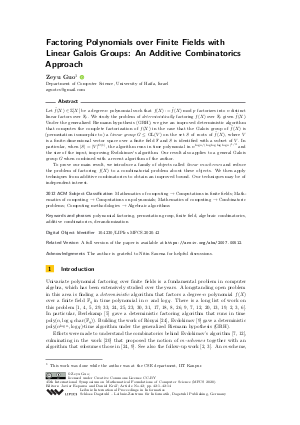Document# Factoring Polynomials over Finite Fields with Linear Galois Groups: An Additive Combinatorics Approach

### Author Zeyu Guo## File

LIPIcs.MFCS.2020.42.pdf
• Filesize: 0.59 MB
• 14 pages

## Acknowledgements

The author is grateful to Nitin Saxena for helpful discussions.

## Cite As

Zeyu Guo. Factoring Polynomials over Finite Fields with Linear Galois Groups: An Additive Combinatorics Approach. In 45th International Symposium on Mathematical Foundations of Computer Science (MFCS 2020). Leibniz International Proceedings in Informatics (LIPIcs), Volume 170, pp. 42:1-42:14, Schloss Dagstuhl - Leibniz-Zentrum für Informatik (2020)
https://doi.org/10.4230/LIPIcs.MFCS.2020.42

## Abstract

Let f̃(X) ∈ ℤ[X] be a degree-n polynomial such that f(X): = f̃(X)od p factorizes into n distinct linear factors over 𝔽_p. We study the problem of deterministically factoring f(X) over 𝔽_p given f̃(X). Under the generalized Riemann hypothesis (GRH), we give an improved deterministic algorithm that computes the complete factorization of f(X) in the case that the Galois group of f̃(X) is (permutation isomorphic to) a linear group G ≤ GL(V) on the set S of roots of f̃(X), where V is a finite-dimensional vector space over a finite field 𝔽 and S is identified with a subset of V. In particular, when |S| = |V|^{Ω(1)}, the algorithm runs in time polynomial in n^{log n/(log log log log n)^{1/3}} and the size of the input, improving Evdokimov’s algorithm. Our result also applies to a general Galois group G when combined with a recent algorithm of the author. To prove our main result, we introduce a family of objects called linear m-schemes and reduce the problem of factoring f(X) to a combinatorial problem about these objects. We then apply techniques from additive combinatorics to obtain an improved bound. Our techniques may be of independent interest.

## Subject Classification

##### ACM Subject Classification
• Mathematics of computing → Computations in finite fields
• Mathematics of computing → Computations on polynomials
• Mathematics of computing → Combinatoric problems
• Computing methodologies → Algebraic algorithms
##### Keywords
• polynomial factoring
• permutation group
• finite field
• algebraic combinatorics
• derandomization

## Metrics

• Access Statistics
• Total Accesses (updated on a weekly basis)
0

## References

1. L. Adleman, K. Manders, and G. Miller. On taking roots in finite fields. In Proceedings of the 18th Annual Symposium on Foundations of Computer Science, pages 175-178, 1977.2. M. Arora. Extensibility of association schemes and GRH-based deterministic polynomial factoring. PhD thesis, Universitäts-und Landesbibliothek Bonn, 2013.3. M. Arora, G. Ivanyos, M. Karpinski, and N. Saxena. Deterministic polynomial factoring and association schemes. LMS Journal of Computation and Mathematics, 17(01):123-140, 2014.4. E. R. Berlekamp. Factoring polynomials over finite fields. Bell System Technical Journal, 46(8):1853-1859, 1967.5. E. R. Berlekamp. Factoring polynomials over large finite fields. Mathematics of Computation, 24(111):713-735, 1970.6. J. Bourgain, S. Konyagin, and I. Shparlinski. Character sums and deterministic polynomial root finding in finite fields. Mathematics of Computation, 84(296):2969-2977, 2015.7. Q. Cheng and M. A. Huang. Factoring polynomials over finite fields and stable colorings of tournaments. In Proceedings of the 4th Algorithmic Number Theory Symposium, pages 233-245, 2000.8. S. A. Evdokimov. Factorization of solvable polynomials over finite fields and the generalized Riemann hypothesis. Journal of Soviet Mathematics, 59(3):842-849, 1992.9. S. A. Evdokimov. Factorization of polynomials over finite fields in subexponential time under GRH. In Proceedings of the 1st Algorithmic Number Theory Symposium, pages 209-219, 1994.10. C. Even-Zohar. On sums of generating sets in ℤ₂ⁿ. Combinatorics, probability and computing, 21(6):916-941, 2012.11. C. Even-Zohar and S. Lovett. The Freiman-Ruzsa theorem over finite fields. Journal of Combinatorial Theory, Series A, 125:333-341, 2014.12. S. Gao. On the deterministic complexity of factoring polynomials. Journal of Symbolic Computation, 31(1):19-36, 2001.13. Y. Guan. Factoring polynomials and Gröbner bases. PhD thesis, Clemson University, 2009.14. Z. Guo. 𝒫-schemes and deterministic polynomial factoring over finite fields. PhD thesis, Caltech, 2017.15. Z. Guo. Deterministic polynomial factoring over finite fields with restricted Galois groups, 2019. Manuscript. URL: https://zeyuguo.bitbucket.io/papers/galois.pdf.
16. Z. Guo. Deterministic polynomial factoring over finite fields: a uniform approach via 𝒫-schemes. Journal of Symbolic Computation, 96:22-61, 2020.17. M. A. Huang. Factorization of polynomials over finite fields and decomposition of primes in algebraic number fields. Journal of Algorithms, 12(3):482-489, 1991.18. M. A. Huang. Generalized Riemann hypothesis and factoring polynomials over finite fields. Journal of Algorithms, 12(3):464-481, 1991.19. G. Ivanyos, M. Karpinski, L. Rónyai, and N. Saxena. Trading GRH for algebra: algorithms for factoring polynomials and related structures. Mathematics of Computation, 81(277):493-531, 2012.20. G. Ivanyos, M. Karpinski, and N. Saxena. Schemes for deterministic polynomial factoring. In Proceedings of the International Symposium on Symbolic and Algebraic Computation, pages 191-198, 2009.21. G. Malle and B. H. Matzat. Inverse Galois Theory. Springer, 1999.22. J. Neukirch. Algebraic Number Theory. Springer-Verlag, 1999.23. J. Pila. Frobenius maps of abelian varieties and finding roots of unity in finite fields. Mathematics of Computation, 55(192):745-763, 1990.24. L. Rónyai. Factoring polynomials over finite fields. Journal of Algorithms, 9(3):391-400, 1988.25. L. Rónyai. Factoring polynomials modulo special primes. Combinatorica, 9(2):199-206, 1989.26. L. Rónyai. Galois groups and factoring polynomials over finite fields. SIAM Journal on Discrete Mathematics, 5(3):345-365, 1992.27. I. Z. Ruzsa. An analog of Freiman’s theorem in groups. Asterisque, 258:323-326, 1999.28. T. Sanders. On the Bogolyubov-Ruzsa lemma. Analysis & PDE, 5(3):627-655, 2012.29. R. Schoof. Elliptic curves over finite fields and the computation of square roots mod p. Mathematics of Computation, 44(170):483-494, 1985.30. V. Shoup. On the deterministic complexity of factoring polynomials over finite fields. Information Processing Letters, 33(5):261-267, 1990.31. V. Shoup. Smoothness and factoring polynomials over finite fields. Information Processing Letters, 38(1):39-42, 1991.32. T. Tao and V. H. Vu. Additive Combinatorics, volume 105. Cambridge University Press, 2006.33. J. von zur Gathen. Factoring polynomials and primitive elements for special primes. Theoretical Computer Science, 52(1):77-89, 1987.34. D. Y. Y. Yun. On square-free decomposition algorithms. In Proceedings of the 3rd ACM Symposium on Symbolic and Algebraic Computation, pages 26-35, 1976.X

Feedback for Dagstuhl Publishing